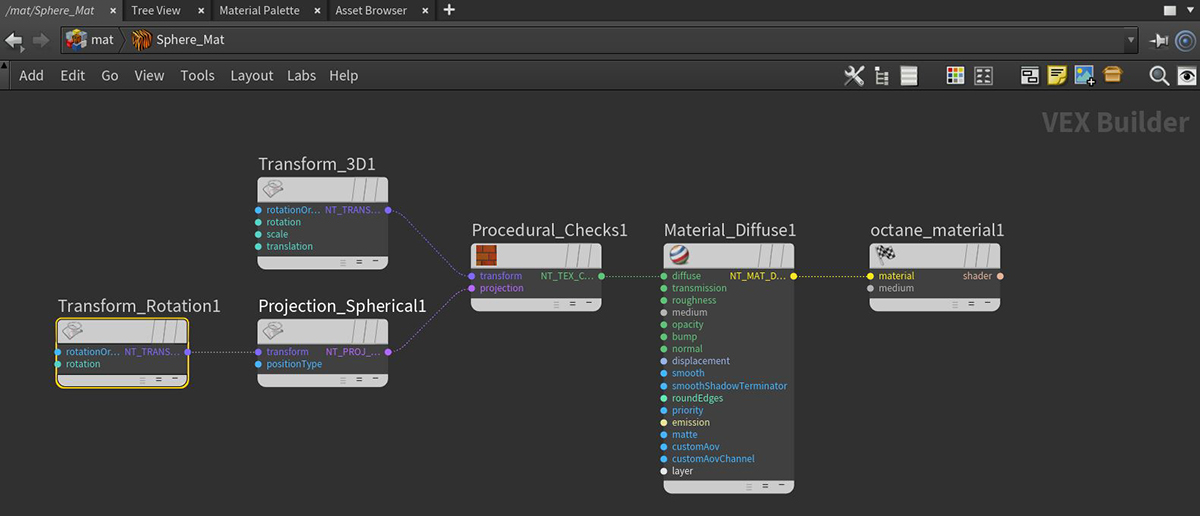# Rotation Transform

The Rotation node modifies a Projection map's rotational data. The same rotational data information is contained in the 3D Transform node, but the Rotation node lets you isolate rotational data where needed (Figure 1).Figure 1: Octane VOP Network example